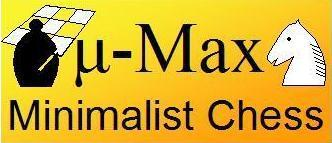## Variable Index

In order to minimize the number of characters in the source code of micro-Max, all variables have one-character names, which makes the names rather non-descriptive. The following list with descriptions might therefore be helpful. Some names are used for different variables in `main()` and `D()`. A letter D or m in the second column of the table distinguishes the case. A space there indicates that this is a global ('static') variable. Most global variables are used to pass information from one instance of the recursive search routine `D()` to the next, or between that routine and the main program. To be visible to the routine that uses them, the corresponding names can then not be used for a local variables. This makes that we have nearly run out of one-letter names for use in `D()`.

In the list below variables used in micro-Max 3.2 or 4.0 are printed in blue. Variables that are already reserved for future enhancements are given in orange.

#### Lower Case

```a  D *_      Pointer to hash-table entry for current position
b    char[]  Chess board as 0x88 array, invalid squares contain piece-square table
c  D
m char[]  In 'main()' this is the input buffer
d  D int     Loop counter of iterative deepening, indicates depth
e  D int     Argument to pass current (differentially updated) evaluation
f  D char    Origin square of threat move
g  D char    Target square of threat move
h  D int     Temporay, used to calculate new remaining ply depth
i  D int     Temporay, used to hold some evaluation terms
j  D int     Loop counter for loop over directions
m int     General loop counter. In Max 4 seed to hash key #2
k  D int     Argument to indicate side to move (8=white, 16=black)
m int     In 'main()' it also holds the side to move
l  D int     Argument to pass upper window limit (beta)
m  D int     Value of best move so far
n    char[]  ASCII representation of pieces on board printout
D int     Argument to pass current (differentially updated) evaluation
o    char[]  list of move vectors, index into this list, and initial-setup array
p  D char    Type of piece doing the move under consideration
m *char   in 'main()' it is a pointer into the input buffer
q  D int     Argument to pass lower window limit (alpha)
r  D int     Step vector of current ray in move generation
s  D
t  D char    Piece to be captured by move under consideration
u  D char    Piece doing the move under consideration
v  D int     Temporay, used to hold some evaluation terms
w    char[]  Relative value of pieces (Pawn = 1)
x  D char    Origin square of move under consideration
y  D char    Target square of move under consideration
z  D int     Argument to pass target square of previous move
```

#### Upper Case

```A    _[]     Hash table
B  D char    Start square of board scan for move generation
C    int     Constant 799 for conversion ASCII -> square number, approx. value queen
D    int()   Recursive search routine
E  D int     Argument to pass e.p. flag F
F  D int     e.p. flag: square skipped over in double move of P or K
G  D int     Corner square for castling, contains S = 0x80 on non-castling
H  D char    Capture square, where captured piece stands
I    int     Constant 8000 = 'infinity' score
J  D int     Argument to pass hash key #1
K    int     Origin square of input move
L    int     Target square of input move
M    int     Constant 0x88 = unused bits in valid square numbers
N    int     Counter of searched nodes, for timing purposes
O    char    Passes e.p. flag to next move at game level
P  D int     Value of null move
Q    int     Passes differentially updated evaluation score to next move
R    char    Origin square of best move returned by 'D()'
S    int     Constant 0x80 = 128-bit (sign bit of char)
T    char[]  Random numbers for hash key, really int, but packed as char
U    MACRO
V    int     Constant 0x70 = rank mask of 0x88 board
W    char    Target square of best move returned by 'D()'
X  D char    Origin square of best move so far
Y  D char    Target square of best move so far, marked with 128-bit as non-castling
Z  D int     Argument to pass hash key #2
_    struct  Name of structure for hash-table entry
```

#### Macro Definitions

```F   Shorthand for for( ; ; )
```_.D   char   Depth